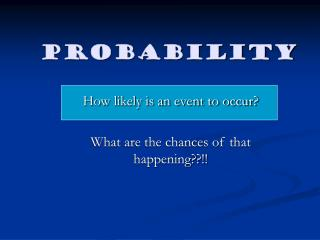# Probability - PowerPoint PPT PresentationDownload PresentationProbability

Probability
Download Presentation## Probability

- - - - - - - - - - - - - - - - - - - - - - - - - - - E N D - - - - - - - - - - - - - - - - - - - - - - - - - - -
##### Presentation Transcript

1. Probability How likely is an event to occur? What are the chances of that happening??!!

2. Lesson objectives: • To understand the terms ‘equal chance’ and ‘outcome’ when thinking about probability. • To complete a maths investigation involving tossing a coin and rolling a dice! - to test the ‘equal chance’ theory!

3. Mathematical Vocabulary • We can describe the probability or chance of an event happening by saying: • It is IMPOSSIBLE. • It is UNLIKELY. • It is LIKELY. • It is CERTAIN.

4. Some events have an equal chance of happening or not happening.Can you think of any?

5. We discussed these: • If you toss a coin you have an equal chance of getting a head or a tail. Heads or tails are the ‘outcomes’. • If a baby is born it has an equal chance of being a boy or a girl. Boy or girl are the ‘outcomes’. • If you roll a dice you have an equal chance of getting the number 1, 2, 3, 4, 5 or 6! These scores are the ‘outcomes’.

6. Today’s task: • We are going to carry out a maths investigation. • We are going to investigate whether we have an equal number of heads and tails when we toss a coin 30 times. • We will also investigate whether we get an equal number of 1s, 2s, 3s, 4, 5s and 6s when we roll a dice 30 times. • We will record our findings.

7. What do you think we will find out? • Perhaps we will have 15 heads and 15 tails when we toss our coin 30 times?? • Perhaps we will have each number on the dice 5 times. (five scores of 1, five scores of 2 etc.) • It’s up to you to find out!!!!

8. Did we achieve out learning objectives today? • We understand what is meant by the terms ‘equal chance’ and ‘outcomes’. • We have carried out an investigation into ‘equal chance’. • We have recorded our findings.

9. Things to think about! • If we rolled 2 coins what possible outcomes could we get? • If we rolled more than 1 dice what possible outcomes could we get? • What would our chances of getting 2 heads or a 6 be like then?!!!